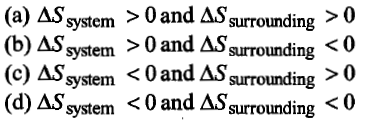When an ideal gas is compressed adiabatically and reversibly, the final temperature is:

(a) higher than the initial temperature

(b) lower than the initial temperature

(c) the same as the initial temperature

(d) dependent on the rate of compression

Concept Questions :-

First Law of Thermodynamics
High Yielding Test Series + Question Bank - NEET 2020

Difficulty Level:

1 litre-atmosphere is equal to:

(a) 101.3 J                           (b) 24.206 cal

(c) 101.3 x 107 erg               (d) all of these

Concept Questions :-

First Law of Thermodynamics
High Yielding Test Series + Question Bank - NEET 2020

Difficulty Level:

The standard change is Gibbs energy for the reaction,

H2O$⇄$H+ + OH- at 25$°$C is:

1. 100 kJ                         2. -90 kJ

3. 90 kJ                           4. -100 kJ

Concept Questions :-

Spontaneity and Entropy
High Yielding Test Series + Question Bank - NEET 2020

Difficulty Level:

The entropy change in the fusion of one mole of a solid melting at 27$°$C (latent heat of fusion is 2930 J mol-1) is

(a) 9.77 JK-1mol-1                (b) 10.73 JK-1mol-1

(c) 2930 JK-1mol-1                (d) 108.5 JK-1mol-1

Concept Questions :-

Spontaneity and Entropy
High Yielding Test Series + Question Bank - NEET 2020

Difficulty Level:

The maximum work done in expanding 16 g oxygen at 300 K and occupying a volume of 5 dm3 isothermally until the volume becomes 25 dm3 is:

(a) 2.01 x 103 J                       (b) +2.81 x 103 J

(c) 2.01 x 10-3 J                      (d) +2.01 x 10-6 J

Concept Questions :-

Internal Energy and work done
High Yielding Test Series + Question Bank - NEET 2020

Difficulty Level:

1 mole of an ideal gas at 25$°\mathrm{C}$ is subjected to expand reversibly ten times of its initial volume. The change in entropy of expansion is:

(a) 19.15 JK-1mol-1                          (b) 16.15 JK-1mol-1

(c) 22.15 JK-1mol-1                          (d) none of these

High Yielding Test Series + Question Bank - NEET 2020

Difficulty Level:

For the process

H2O(l) $\to$H2O(g)

at T=100$°$C and 1 atmosphere pressure, the correct choice is:Concept Questions :-

Spontaneity and Entropy
High Yielding Test Series + Question Bank - NEET 2020

Difficulty Level:

(a) pressure is maintained constant

(b) gas is isothermally expanded

(c) there is perfect heat insulation

(d) the system changes heat with surroundings

Concept Questions :-

Internal Energy and work done
High Yielding Test Series + Question Bank - NEET 2020

Difficulty Level:

Heat of combustion $∆\mathrm{H}°$ for C(s), H2(g) and CH4(g) are -94, -68 and -213 kcal/mol. Then, $∆\mathrm{H}°$ for

C(s) + 2H2(g) $\to$CH4(g) is

(a) -17 kcal/mol                          (b) -111 kcal/mol

(c) -170 kcal/mol                        (d) -85 kcal/mol

Concept Questions :-

Thermochemistry
High Yielding Test Series + Question Bank - NEET 2020

Difficulty Level:

If 50 calorie are added to a system and system does work of 30 calorie on surroundings, the change in internal energy of system is:

(a) 20 cal                             (b) 50 cal

(c) 40 cal                             (d) 30 cal

Concept Questions :-

Enthalpy and It's Type